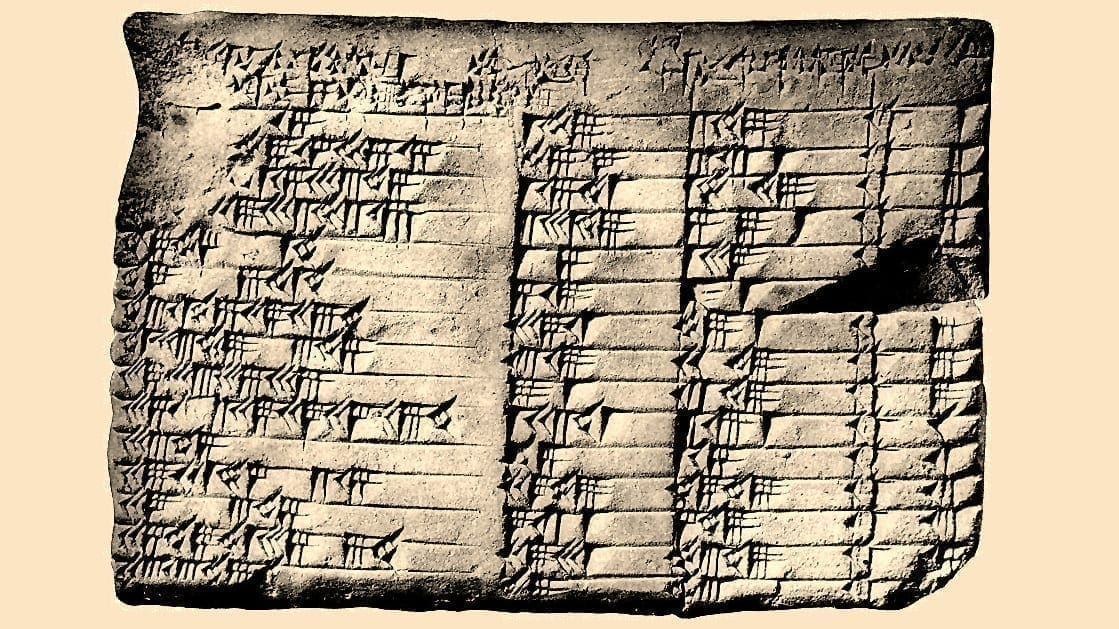# Trigonometry Around the Time of Abraham[Originally published as Trigonometry from the 18th Century BC]

Back in 1922, G.A. Plimpton bought the tablet shown above from an archaeologist named Edgar Banks, and it has become known as “Plimpton 322.” According to an analysis of the writing, it is of Babylonian origin and probably dates back to the 18th century BC. It has been known for a while that Plimpton 322 is a mathematical table that contains ratios related to triangles. However, there were aspects of the table that didn’t make sense, at least until recently. According to a study published in Historia Mathematica, it is actually the world’s oldest trigonometry table!

For those of you who didn’t take (or don’t remember) trigonometry, it is a branch of mathematics that deals with triangles. I was first introduced to it in high school, as part of my “college preparatory” mathematics education. One thing that initially struck me about this branch of mathematics was the fact that there were times you had to use a lookup table (or a calculator) in order to get the results you needed. I had never before done math like that. Sure, calculators made some math faster and certainly cut down on errors. However, for some trigonometry problems, you simply couldn’t get the answer without looking up numbers in a table or using a calculator.

Once I studied chemistry and physics at university, trigonometry became a pretty constant companion. In physics, you use it to analyze vectors, which are one of the most fundamental aspects of that scientific discipline. In chemistry, you use it to study molecular structure. Over time, I got really adept at using my calculator to solve trigonometry-related problems. Interestingly enough, however, this tablet represents a completely different means by which you can do trigonometry.

In the trigonometry we use today, angles are important. However, in this Babylonian trigonometry, angles aren’t used. Instead, only the lengths of a triangle’s sides are used. Indeed, in figuring out what this tablet means, the authors state:

First we abandon the notion of angle, and instead describe a right triangle in terms of the short side, long side and diagonal of a rectangle.

I am sure it is easy for mathematicians to do such a thing, but it is hard for me to wrap my head around the idea that you can do trigonometry without angles. However, they show that when you look at the tablet that way, it is clearly a lookup table that gives you everything you need to make specific trigonometry calculations. Why would the Babylonians be using such a table? The authors refuse to speculate, but most likely, the Babylonians were using trigonometry to survey fields and build structures like temples and palaces.

There are two other really interesting things about this table. First, it predates what historians thought was the earliest form of trigonometry, which was developed by the Greek mathematician Hipparchus in the second century BC. That means the Babylonians, not the Greeks, can be credited as the first to investigate this mathematical discipline.

Second, this roughly 3,700-year-old tablet represents in some ways an improvement on the trigonometry that we do. That’s because our trigonometry, which is based on lookup tables and calculators, is really an approximation.

For example, if the answer is one-third, a lookup table or a calculator will usually have something like 0.3333. While that’s an excellent approximation of one-third, it is not exact. Only the fraction 1/3 is an exact representation of one-third. It turns out that this table has only exact representations. While there is no practical difference between a really good approximation and an exact value, from a mathematical point of view, an exact value is more elegant. So if nothing else, this table is more mathematically elegant than our modern tables and calculators.

If you’ve ever had the misguided notion that ancient people were “ignorant savages,” finds like this one should make you change your mind!

## Written by Jay Wile

As a scientist, it is hard for me to fathom anyone who has scientific training and does not believe in God. Indeed, it was science that brought me not only to a belief in God, but also to faith in Christianity. I have an earned Ph.D. from the University of Rochester in nuclear chemistry and a B.S. in chemistry from the same institution. blog.drwile.com